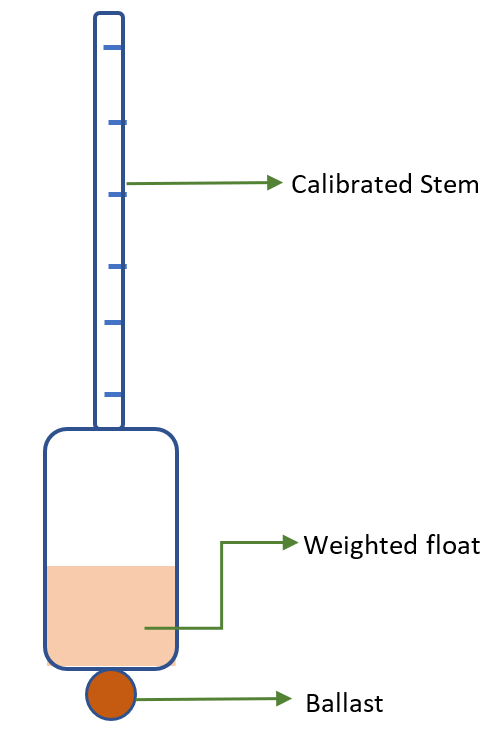A hydrometer is used to measure which of the following quantities of a liquid?A. MassB. VolumeC. Relative densityD. TemperatureVerified
145.8k+ views
Hint: The quantity that it measures is sometimes referred to as specific gravity. This quantity does not have any units and is just a ratio. The device however uses the principle of fluid displacement to determine this quantity.

Let us first define what a hydrometer is, what quantity it measures and how it does so.A hydrometer is a sealed, hollow glass device that is used to measure the relative density or the specific gravity of a liquid and is based on the concept of buoyancy.

Relative density is the ratio of the density of a substance to the density of a given reference material. The reference material is what is filled in the weighted float.

The structure of the hydrometer consists of two parts:

(i)Calibrated stem: It is the part of the hydrometer from which we obtain the readings. The measuring scale is usually calibrated to the reference material in the float.
(ii)The float: It has a broad base to facilitate buoyancy, a ballast such as lead or mercury to maintain stability, and a weight that is filled inside the float, which is most often the reference material.

Let us see how it works:

The fluid whose relative density we have to test is poured in a tall container and the hydrometer is gently lowered into the fluid until it is able to float freely by itself. The point at which the fluid surface touches the stem of the hydrometer is indicative of its relative density.
Therefore, a hydrometer sinks deeper in low-density liquids and less deep in high-density liquids.

So, the correct answer is “Option C”.

Note:
Note that ultimately, the hydrometer makes use of the Archimedes’ principle which says that an object immersed in a fluid experiences an upward buoyant force that is equal in magnitude to the weight of the displaced fluid. By observing the extent of this buoyancy, we are able to determine the relative density of the fluid.